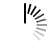## Java.util.Scanner.remove() Method

```package com.tutorialspoint;

import java.util.*;

public class ScannerDemo {
public static void main(String[] args) {

String s = "Hello World! 3 + 3.0 = 6.0 true ";

// create a new scanner with the specified String Object
Scanner scanner = new Scanner(s);

// print a line of the scanner
System.out.println("" + scanner.nextLine());

// attempt to call remove results in an exception
scanner.remove();

// close the scanner
scanner.close();
}
}```

```package com.tutorialspoint;

import java.util.*;

public class ScannerDemo {
public static void main(String[] args) {

String s = "Hello World! 3 + 3.0 = 6.0 true ";

// create a new scanner with the specified String Object
Scanner scanner = new Scanner(s);

// print a line of the scanner
System.out.println("" + scanner.nextLine());

// print the default radix

// close the scanner
scanner.close();
}
}```

## Java.util.Scanner.nextShort(int radix) Method

```package com.tutorialspoint;

import java.util.*;

public class ScannerDemo {
public static void main(String[] args) {

String s = "Hello World! 3 + 3.0 = 6.0 true ";

// create a new scanner with the specified String Object
Scanner scanner = new Scanner(s);

// find the next short token and print it
// loop for the whole scanner
while (scanner.hasNext()) {

// if the next is a short, print found and the short with radix 4
if (scanner.hasNextShort()) {
System.out.println("Found :" + scanner.nextShort(4));
}

// if no short is found, print "Not Found:" and the token
}

// close the scanner
scanner.close();
}
}```

## Java.util.Scanner.nextShort() Method

```package com.tutorialspoint;

import java.util.*;

public class ScannerDemo {
public static void main(String[] args) {

String s = "Hello World! 3 + 3.0 = 6.0 true ";

// create a new scanner with the specified String Object
Scanner scanner = new Scanner(s);

// find the next short token and print it
// loop for the whole scanner
while (scanner.hasNext()) {

// if the next is a short, print found and the short
if (scanner.hasNextShort()) {
System.out.println("Found :" + scanner.nextShort());
}

// if no short is found, print "Not Found:" and the token
}

// close the scanner
scanner.close();
}
}```

## Java.util.Scanner.nextLong(int radix) Method

```package com.tutorialspoint;

import java.util.*;

public class ScannerDemo {
public static void main(String[] args) {

String s = "Hello World! 3 + 3.0 = 6.0 true ";
Long l = 13964599874l;
s = s + l;

// create a new scanner with the specified String Object
Scanner scanner = new Scanner(s);

// find the next long token and print it
// loop for the whole scanner
while (scanner.hasNext()) {

// if no long is found, print "Not Found:" and the token

// if the next is a long, print found and the long with radix 20
if (scanner.hasNextLong()) {
System.out.println("Found :" + scanner.nextLong(20));
}
}

// close the scanner
scanner.close();
}
}```

## Java.util.Scanner.nextLong() Method

```package com.tutorialspoint;

import java.util.*;

public class ScannerDemo {
public static void main(String[] args) {

String s = "Hello World! 3 + 3.0 = 6.0 true ";
Long l = 13964599874l;
s = s + l;

// create a new scanner with the specified String Object
Scanner scanner = new Scanner(s);

// find the next long token and print it
// loop for the whole scanner
while (scanner.hasNext()) {

// if no long is found, print "Not Found:" and the token

// if the next is a long, print found and the long
if (scanner.hasNextLong()) {
System.out.println("Found :" + scanner.nextLong());
}
}

// close the scanner
scanner.close();
}
}```

## Java.util.Scanner.nextLine() Method

```package com.tutorialspoint;

import java.util.*;

public class ScannerDemo {
public static void main(String[] args) {

String s = "Hello World! \n 3 + 3.0 = 6.0 true ";

// create a new scanner with the specified String Object
Scanner scanner = new Scanner(s);

// print the next line
System.out.println("" + scanner.nextLine());

// print the next line again
System.out.println("" + scanner.nextLine());

// close the scanner
scanner.close();
}
}```

## Java.util.Scanner.nextInt(int radix) Method

```package com.tutorialspoint;

import java.util.*;

public class ScannerDemo {
public static void main(String[] args) {

String s = "Hello World! 3 + 3.0 = 6.0 true ";

// create a new scanner with the specified String Object
Scanner scanner = new Scanner(s);

// find the next int token and print it
// loop for the whole scanner
while (scanner.hasNext()) {

// if the next is a int, print found and the int with radix 4
if (scanner.hasNextInt()) {
System.out.println("Found :" + scanner.nextInt(4));
}
// if no int is found, print "Not Found:" and the token
}

// close the scanner
scanner.close();
}
}```

## Java.util.Scanner.nextInt() Method

```package com.tutorialspoint;

import java.util.*;

public class ScannerDemo {
public static void main(String[] args) {

String s = "Hello World! 3 + 3.0 = 6.0 true ";

// create a new scanner with the specified String Object
Scanner scanner = new Scanner(s);

// find the next int token and print it
// loop for the whole scanner
while (scanner.hasNext()) {

// if the next is a int, print found and the int
if (scanner.hasNextInt()) {
System.out.println("Found :" + scanner.nextInt());
}
// if no int is found, print "Not Found:" and the token
}

// close the scanner
scanner.close();
}
}```

## Java.util.Scanner.nextFloat() Method

```package com.tutorialspoint;

import java.util.*;

public class ScannerDemo {
public static void main(String[] args) {

String s = "Hello World! 3 + 3.0 = 6.0 true ";
Float f = 1.2385f;
s = s + f;

// create a new scanner with the specified String Object
Scanner scanner = new Scanner(s);

// use US locale to be able to identify floats in the string
scanner.useLocale(Locale.US);

// find the next float token and print it
// loop for the whole scanner
while (scanner.hasNext()) {
scanner.next();

// if the next is a float, print found and the float
if (scanner.hasNextFloat()) {
System.out.println("Found :" + scanner.nextFloat());
}
}

// close the scanner
scanner.close();
}
}```